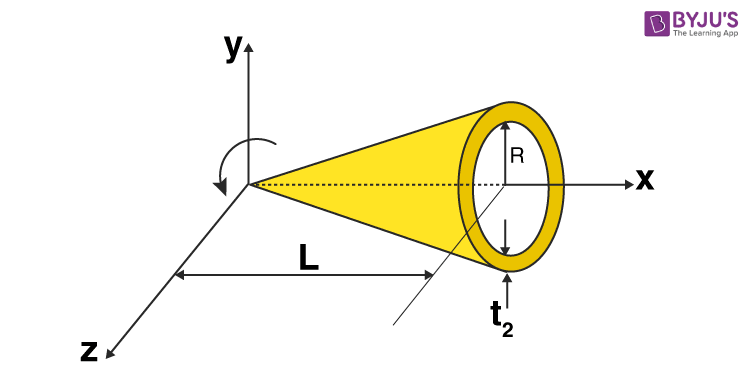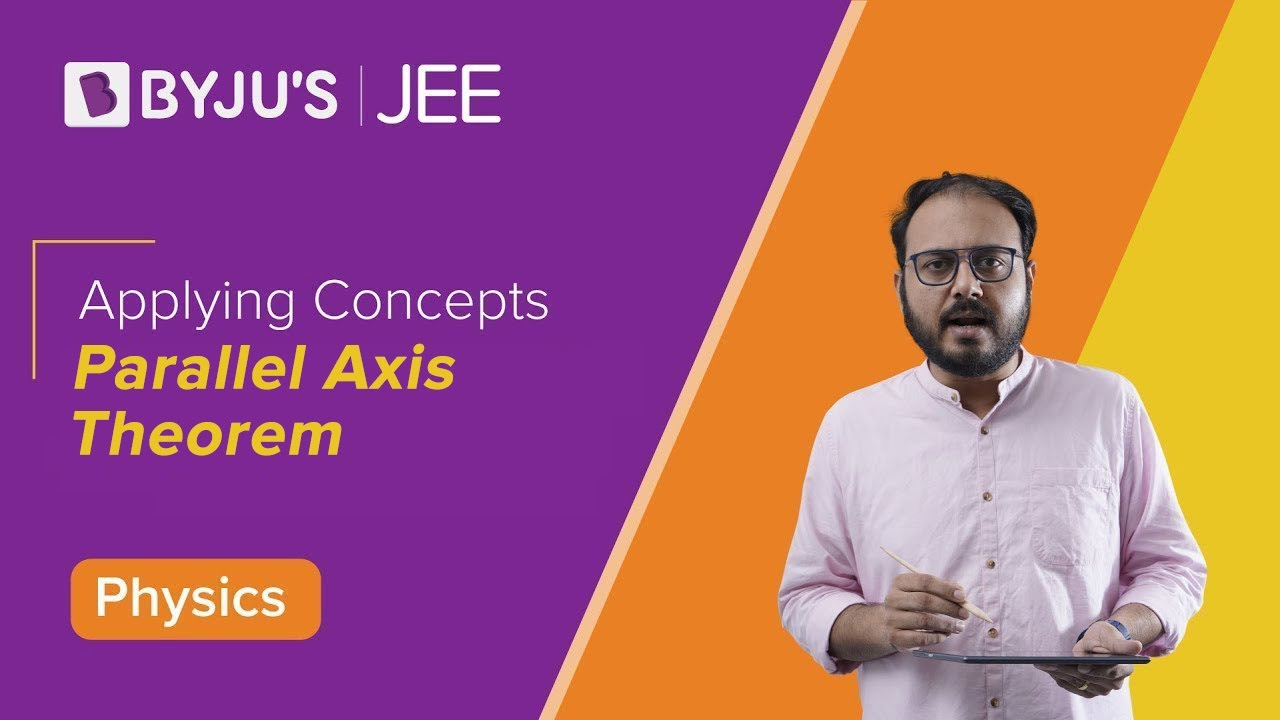Checkout JEE MAINS 2022 Question Paper Analysis : Checkout JEE MAINS 2022 Question Paper Analysis :

# Moment Of Inertia Of Hollow Cone

Moment of inertia of hollow cone can be determined using the following expression;

 I = MR2 / 2

Here students will learn and understand how the formula is derived as well as its application.

## Moment Of Inertia Of Hollow Cone Formula Derivation

For deriving the moment of inertia formula of a hollow cone we can basically follow some general guidelines which are;

• Determining the mass density in terms of per unit area.
• Defining parameters and finding the mass of small parts.
• Finding the moment of inertia of the small parts corresponding to a ring.
• Integration1. There is a hollow cone with radius R, height H and mass M.

Now, we will take the element disc at a slant height l and | radius r having thickness dl and mass dm.

Using the similarity of the triangle we get;

$$\begin{array}{l}\frac{r}{x}= \frac{R}{\sqrt{R^{2}+H^{2}}}\end{array}$$

$$\begin{array}{l}r= \frac{R}{\sqrt{R^{2}+H^{2}}}x\end{array}$$

The mass of the elemental disc mass is given by;

$$\begin{array}{l}dm = \frac{M}{\pi R\sqrt{R^{2}+H^{2}}}2\pi rdx\end{array}$$

$$\begin{array}{l}dm = \frac{M}{\pi R\sqrt{R^{2}+H^{2}}}2\pi \frac{R}{\sqrt{R^{2}+H^{2}}}xdx\end{array}$$

$$\begin{array}{l}dm = \frac{2Mxdx}{R^{2}+H^{2}}\end{array}$$

2. Calculating the moment of inertia of the elemental disk. It will be given as;

Moment of inertia of element is, dI = dmr2

$$\begin{array}{l}dI= \frac{2Mxdx}{R^{2}+H^{2}}\frac{R^{2}}{{R^{2}+H^{2}}}x^{2}\end{array}$$

3. Finding the moment of inertia through integration.

$$\begin{array}{l}I= \int \frac{2Mxdx}{R^{2}+H^{2}}\frac{R^{2}}{{R^{2}+H^{2}}}x^{2}dx\end{array}$$

I = [2MR2 / (R2 + H2)2]∫ x3dx

Using the limits of x where x usually varies from o to

$$\begin{array}{l}\sqrt{R^{2}+H^{2}}\end{array}$$

We now get;

I = [2MR2 / (R2 + H2)2 ] x [(R2 + H2)2 / 4]

I = ½ MR2

Therefore, I = MR2 / 2

## Parallel Axis Theorem